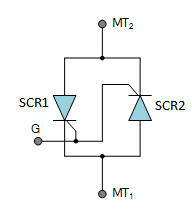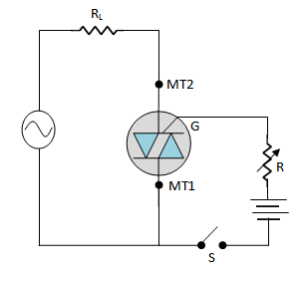# Construction and working of Triac

## Construction and working of Triac

In this article we will explain the construction and working of triac in detail.

### The Triac

The major drawback of an SCR is that it can conduct current in one direction only. Therefore, an SCR can only control d.c. power or forward biased half-cycles of a.c. in a load. However, in an a.c. system, it is often desirable and necessary to exercise control over both positive and negative half-cycles. For this purpose, a semiconductor device called triac is used.

A triac is a three-terminal semiconductor switching device which can control alternating current in a load.

Triac is an abbreviation for triode a.c. switch. ‘Tri’ – indicated that the device has three terminalsand ‘ac’ means that the device controls alternating current or can conduct current in either direction.

Function of a triac can be understood by referring to the simplified fig.1. The control circuit of triac can be adjusted to pass the desired portions of positive and negative half-cycle of a.c. supply through the load RL.

(i)                                                                                                               (ii)

Fig.1

Thus, referring to fig.1(ii), the triac passes the positive half-cycle of the supply from Θto 180i.e. the shaded portion of positive half-cycle.

Similarly, the shaded portion of negative half-cycle will pass through the load. In this way, the alternating current and hence a.c. power flowing through the load can be controlled.

### Triac Construction

A triac is a three-terminal, five layer semiconductor device whose forward and reverse characteristics are identical to the forward characteristics of the SCR.

The three terminals are designated as main terminal MT1, main terminal MT2  and gate G.

Fig.2 (i) shows the basic structure of a triac.

(i)                                                                            (ii)                                                                        (iii)

Fig.2

A triac is equivalent to two separate SCRs connected in inverse parallel i.e. anode of each connected to the cathode of the other, with gates commoned as shown in fig.2 (ii).

Therefore, a triac acts like a bidirectional switch i.e. it can conduct current in either direction.

Fig.2 (iii) shows the schematic symbol of a triac. The symbol consists of two parallel diodes connected in opposite directions with a single gate lead. The gate provides control over conduction in either direction.

The foll0wing points may be noted about the triac:

1. The triac can conduct current with proper gate current regardless of the polarities of the main terminals MT1 and MT2. Since there is no longer a specific anode or cathode, the main leads are referred to as MTand MT2.
2. A triac can be turned on either with a positive or negative voltage at the gate of the device.
3. Like the SCR, once the triac is fired into conduction, the gate loses all control. The triac can be turned off by reducing the circuit current to the value of holding current.
4. The main disadvantage of triac over SCRs is that triacs have considerably lower current handling capabilities. Most triacs are available in ratings of less than 40A at voltage up to 600V.

#### SCR Equivalent Circuit of Triac

A triac is equivalent to two separate SCRs connected in inverse  connected in inverse parallel i.e. anode of each connected to the cathode of the other with gates commoned.

Fig.3 (i) shows the basic structure of a triac.

(i)                                                                                                                          (ii)

Fig.3

If we split the basic structure of a triac into two halves as shown in fig.3 (ii), we can see that we have two SCRs connected in inverse parallel.

The left half in fig.3(ii) consists of a pnpn device(p1n2p2n4) having three pn junctions and constitutes SCR1.

Similarly, the right half in fig.3 (ii) consists of pnpn device (p2n3p1n1) having three pn junctions and constitutes SCR2.

The SCR equivalent circuit of the triac is shown in fig.4.Fig.4

Suppose the main terminal MTis positive and main terminal MTis negative.

If the triac is now fired into conduction by proper gate current, the triac will conduct current following the path (left half) shown in fig.3(ii).

In relation to fig.4, the SCR1 is ON and the SCR2 if OFF.

Now suppose that MTis negative and MTis positive.

With proper gate current, the triac will be fired into conduction and current through the device follows the path (right half) as shown in fig.3(ii).

In relation to fig.4, the SCR2 is ON and SCR1 is OFF.

#### Triac Operation

Fig.5 shows the simple triac circuit.Fig.5

The a.c. supply to be controlled is connected across the main terminals of the triac through a load resistance RL.

The gate circuit consists of battery, a current limiting resistor R and a switch S.

The circuit action is as follows :

1.  With switch S open, there will be no gate current and the triac is cut off.

Even with no gate current, the triac can be turned on provided the supply voltage becomes equal to the breakover voltage of triac.

However, the normal way to turn on a triac is by introducing a proper gate current.

2.  When switch S is closedthe gate current starts flowing in the gate cirucit.

Ina  similar manner to SCR, the breakover voltage of the triac can be varied by making proper gate current to flow.

With a few  milliamperes introduced at the gate, the triac will start conducting whether terminal MTis positive or negative w.r.t. MT.

3.   If terminal MTis positive w.r.t.  MT, the tria turns on and the conventional current will flow from MTto MT1.

If the terminal MTis negative w.r.t. MT, the triac is again turned on but this time the conventional current flows from MT1 to MT2.

The above action of triac reveals that it can act as an a.c. contactor to switch on or off alternating current to a load.

The additional advantage of triac is that by adjusting the gate current to a proper value, any portion of both positive and negative half cycles of a.c. supply can be made to flow through the load. This permits to adjust the transfer of a.c. power from the source to the load.